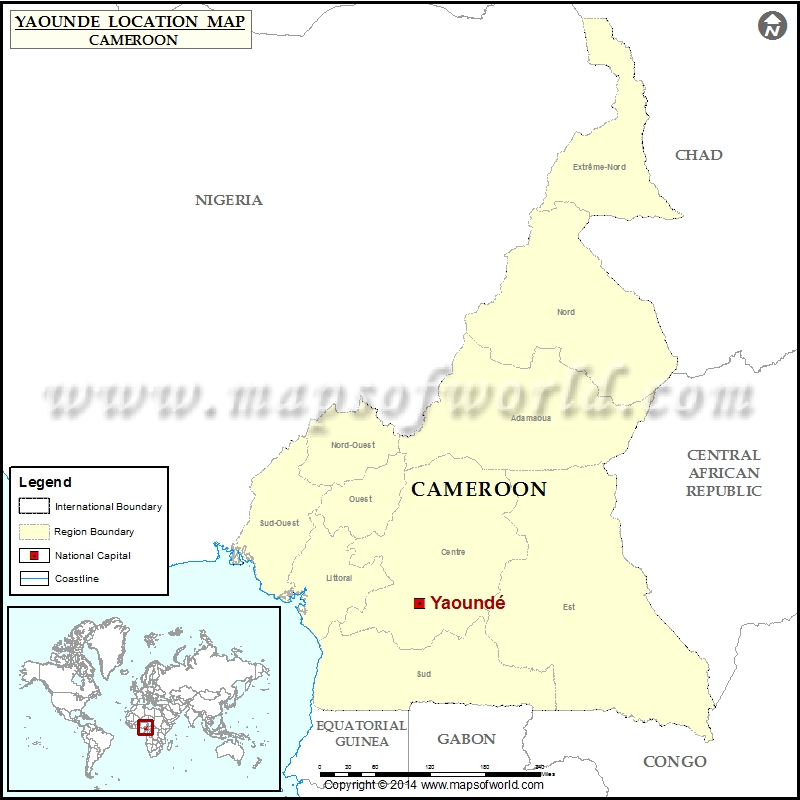﻿ Where is Yaounde | Location of Yaounde in Cameroon Map

# Where is YaoundeDescription :  Map showing location of Yaounde in Cameroon. Disclaimer

 Close Disclaimer : All efforts have been made to make this image accurate. However Compare Infobase Limited,its directors and employees do not own any responsibility for the correctness or authenticity of the same.

Search For Latitude & Longitude Map

By Country

Or

Latitude

Longitude

Or

Area

* Put South & West values with minus ( - ) sign.

City NameYaounde
ContinentAfrica
CountryCameroon
StateCentre
Area180 km2 (70 sq mi)
Population2,440,462 (2012)
Lat Long3.86905,11.522498
Official LanguageEnglish and French
Area Code(+237) 3
Time ZoneCET (UTC+1)
Airport Yaounde Nsimalen International Airport
Major AttractionsYaounde cathedral, Cameroon National Museum, Cameroon Art Museum
Major RiversCameroon river
 Location Maps of Cities in Cameroon
 Where is Abong-Mbang Where is Ako Where is Akonolinga Where is Ambam Where is Bafang Where is Bafia Where is Bafoussam Where is Bafut Where is Bagangte Where is Bali Where is Bamenda Where is Bamingie Where is Bandjoun Where is Bangem Where is Banyo Where is Batouri Where is Belabo Where is Bertoua Where is Buea Where is Campo Where is Dimako Where is Dizangue Where is Djoum Where is Douala Where is Dschang Where is Ebolowa Where is Edea Where is Foumban Where is Foumbot Where is Garoua Where is Goura Where is Guider Where is Idenau Where is Kaele Where is Kousseri Where is Kribi Where is Kumba Where is Kumbo Where is Limbe Where is Lomie Where is Loum Where is Makenene Where is Mamfe Where is Maroua Where is Martap Where is Mbalmayo Where is Mbandjock Where is Mbengwi Where is Mbouda Where is Meiganga Where is Melong Where is Menji Where is Minam Where is Mokolo Where is Monatele Where is Mora Where is Mouloudou Where is Mutengene Where is Ndu Where is Ngaoundere Where is Nkambe Where is Nkongsamba Where is Saa Where is Sangmelima Where is Tibati Where is Tiko Where is Wum Where is Yagoua Where is Yaounde Where is Yokadouma